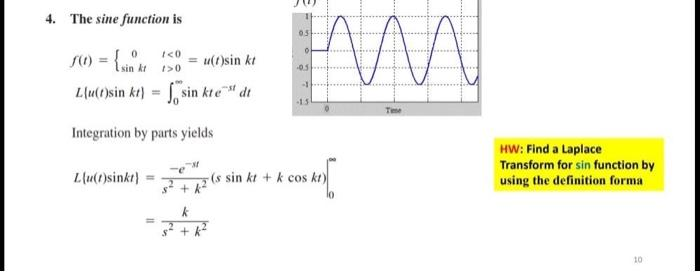### Create an Account

Already have account?

### Forgot Your Password ?

Home / Questions / 4. The sine function is 031 0 (0) = { sin ICO = u(d)sin ki 05 -1 Z(u(t)sin kt) sin kte" di...

# 4. The sine function is 031 0 (0) = { sin ICO = u(d)sin ki 05 -1 Z(u(t)sin kt) sin kte" dit 15 Integration by parts yields HW: Find a Laplace Transform for sin function by using the definition forma L

4. The sine function is 031 0 (0) = { sin ICO = u(d)sin ki 05 -1 Z(u(t)sin kt) sin kte" dit 15 Integration by parts yields HW: Find a Laplace Transform for sin function by using the definition forma Llu(i)sinki) (s sin kt + k cos ko) ab + k 10Apr 15 2021 View more View Less

#### Answer (Solved)Subscribe To Get Solution